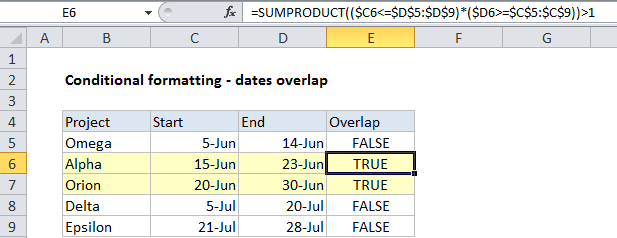# Conditional formatting dates overlap in Excel

This tutorial shows how to work Conditional formatting dates overlap in Excel using the example below;

## Formula

`=SUMPRODUCT((start_date<=end_dates)*(end_date>=start_dates))>1`## Explanation

To highlight cells where dates overlap you can use conditional formatting with a formula based on the SUMPRODUCT function. In the example shown the formula in south E6 is:

`=SUMPRODUCT((\$C6<=\$D\$5:\$D\$9)*(\$D6>=\$C\$5:\$C\$9))>1`

This is the same formula used to highlight entire rows in the table using a formula-based conditional formatting rule.

Also See:   Sum if equal to one of many things in Excel

### How this formula works

Consider for a moment how overlapping dates work. For a project to overlap the dates of other projects, two conditions must be true:

1. The start date must be less than or equal (<=) to at least one other end date and the list.

2. The end date for the project must be greater than or equal to (>=) at least one other start date in the list.

Also See:   Count cells that contain errors in Excel

If both of these conditions are true, the project dates must overlap another project in that list.

The SUMPRODUCT function is perfect for this kind of test because it handles array comparisons elegantly.

To check a project start date against all end dates, we use this expression:

`(\$C6<=\$D\$5:\$D\$9)`

To check a project end date against all end dates, we use this expression:

`(\$D6>=\$C\$5:\$C\$9)`

The resulting arrays of TRUE FALSE values are multiplied by each other inside SUMPRODUCT. This coerces the TRUE and FALSE results into 1s and 0s automatically, so the formula is solved like this:

```=SUMPRODUCT({0;1;1;1;1}*{1;1;1;0;0})>1
=SUMPRODUCT({0;1;1;0;0})>1
=TRUE```